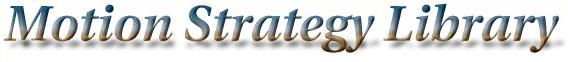Main Page   Class Hierarchy   Compound List   File List   Compound Members   File Members

# Model3DRigidTree Class Reference

A 3D kinematic tree of bodies that uses DH parameters. More...

#include <model3d.h>

Inheritance diagram for Model3DRigidTree::List of all members.

## Public Methods

Model3DRigidTree (string path)
virtual ~Model3DRigidTree ()
virtual MSLVector StateTransitionEquation (const MSLVector &x, const MSLVector &u)
The state transition equation, or equations of motion, xdot=f(x,u).

virtual MSLVector StateToConfiguration (const MSLVector &x)
A method that converts a Model state in to a Geom configuration.

virtual MSLVector LinearInterpolate (const MSLVector &x1, const MSLVector &x2, const double &a)
Linearly interpolate two state while respecting topology. More...

virtual double Metric (const MSLVector &x1, const MSLVector &x2)
A distance metric, which is Euclidean in the base class.

virtual bool Satisfied (const MSLVector &x)
Test whether global state-space constraints are satisfied.

## Public Attributes

int NumBodies
Number of bodies in the tree.

MSLVector DH
The distances between joints ("a" parameters in kinematics).

vector<int> StateIndices
vector<int> Parents

## Detailed Description

A 3D kinematic tree of bodies that uses DH parameters.

A 3D kinematic tree of bodies that uses DH parameters which should be given in a DH file in the following order: Alpha(1,2...), Theta(1,2...), A(1,2...), D(1,2...). Some of these parameters change during the movement, and each of these parameters becomes a state variable. The indices of these parameters should be given in the file StateIndices (i.e., each entry corresponds to a state, and indicates which DH parameter is variable). For each body in the tree, the parent body in the tree should be indicated. This is specified in the file Parents. Each entry in Parents corresponds to a body (the i^th element is Robot i). The first entry is the root (this index is ignored because the root has no parent). The bodies in the tree must be arranged so that the indices in Parents are in nondecrreasing order (corresponding, for example to a depth-first search of the tree).

## Constructor & Destructor Documentation

 Model3DRigidTree::Model3DRigidTree ( string path )

 Model3DRigidTree::~Model3DRigidTree ( ) [inline, virtual]

## Member Function Documentation

 virtual MSLVector Model3DRigidTree::LinearInterpolate ( const MSLVector & x1, const MSLVector & x2, const double & a ) [virtual]
 Linearly interpolate two state while respecting topology. If a=0, then x1 is returned; if a=1, then x2 is returned. All intermediate values of $a \in [0,1]$ yield intermediate states. This method is defined by Model. Reimplemented from Model3DRigid.

 virtual double Model3DRigidTree::Metric ( const MSLVector & x1, const MSLVector & x2 ) [virtual]
 A distance metric, which is Euclidean in the base class. Reimplemented from Model3DRigid.

 virtual bool Model3DRigidTree::Satisfied ( const MSLVector & x ) [virtual]
 Test whether global state-space constraints are satisfied. Reimplemented from Model.

 virtual MSLVector Model3DRigidTree::StateToConfiguration ( const MSLVector & x ) [virtual]
 A method that converts a Model state in to a Geom configuration. Reimplemented from Model.

 virtual MSLVector Model3DRigidTree::StateTransitionEquation ( const MSLVector & x, const MSLVector & u ) [virtual]
 The state transition equation, or equations of motion, xdot=f(x,u). Reimplemented from Model3DRigid.

## Member Data Documentation

 MSLVector Model3DRigidTree::DH
 The distances between joints ("a" parameters in kinematics).

 int Model3DRigidTree::NumBodies
 Number of bodies in the tree.

 vector Model3DRigidTree::Parents

 vector Model3DRigidTree::StateIndices

The documentation for this class was generated from the following file: Motion Strategy Library

Web page maintained by Steve LaValle
Partial support provided by NSF CAREER Award IRI-970228 (LaValle), Honda Research.
Contributors: Anna Atramentov, Peng Cheng, James Kuffner, Steve LaValle, and Libo Yang.